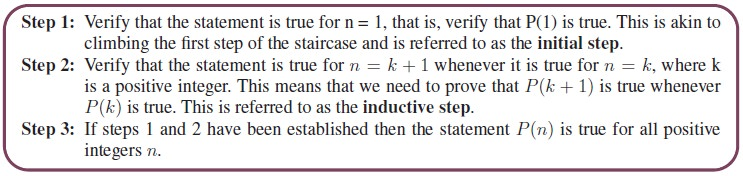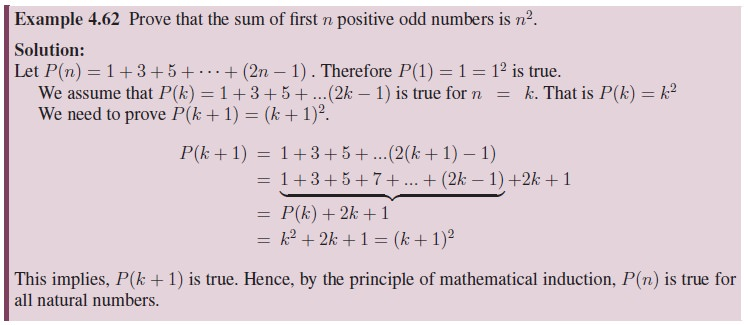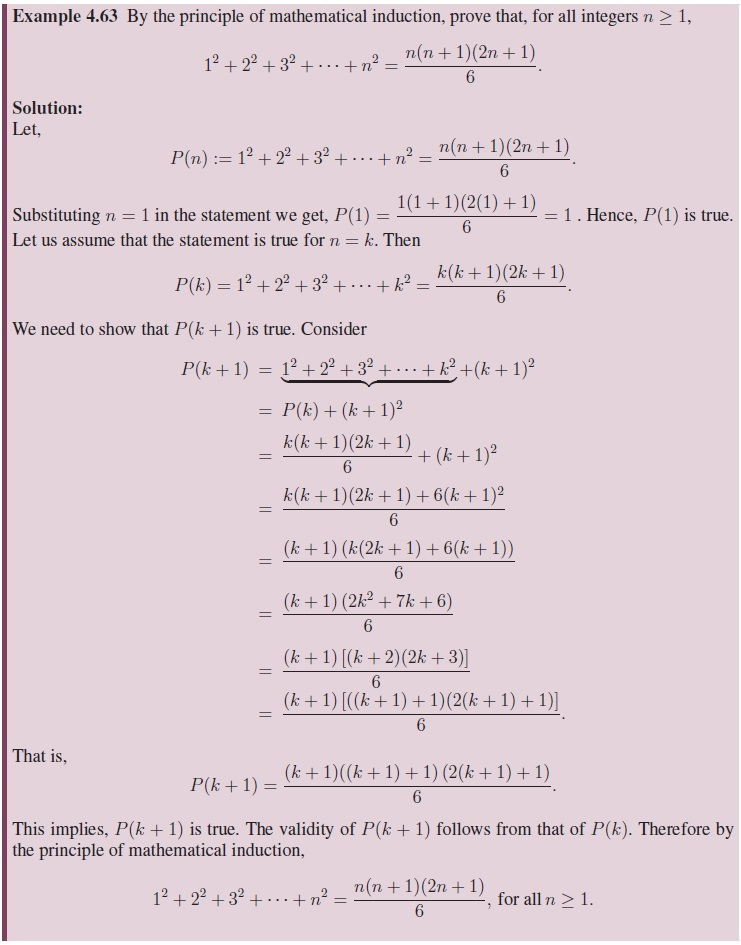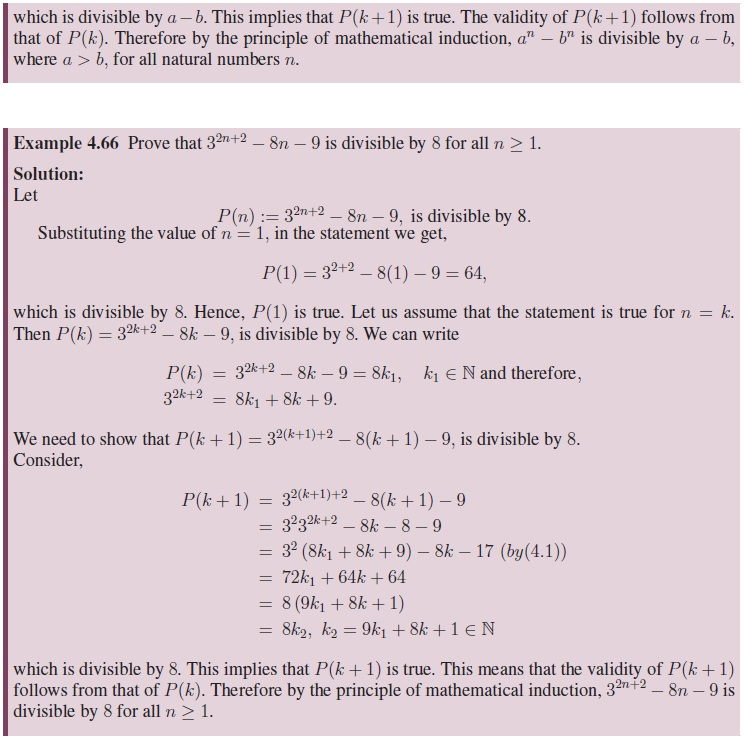Home | | Maths 11th std | Mathematical induction

# Mathematical induction

Let us consider the sum of the first n positive odd numbers. These are 1, 3, 5, 7, • • • , 2n − 1. The first odd number 1 which is equal to 1.

Mathematical induction

Let us consider the sum of the first n positive odd numbers. These are 1, 3, 5, 7, · · · , 2n 1. The first odd number 1 which is equal to 1. The first two odd numbers are 1 and 3 and their sum is 4. Writing these as follows helps us to see a pattern.and so on. We note that the right hand side of the expressions are the perfect squares 1, 4, 9, 16, 25 · · · . This pattern compels us to make the conjecture that the sum of the first n odd numbers is equal to n2. Symbolically, we express this as,

1 + 3 + 5 + · · · + (2n 1) = n2.

However we have only made a conjecture. In order to prove the conjecture we shall use the Principle of Mathematical Induction. Mathematical Induction is a method or technique of proving mathematical results or theorems of the above kind. This technique relies upon making conjectures by observing all possible cases of a specific result. It is well suited for proving results in algebra or in other disciplines of mathematics where results or theorems are stated in terms of n, n being a positive integer. The process of Mathematical Induction may be compared to that of climbing an infinite staircase.

In order to ensure that we complete the climb, it is sufficient to ensure the following.

a.     We can climb the first step.

b.     Once we have reached a particular step of the staircase, we can climb to the next step.

Being sure of (a) and (b) will enable us to climb all the steps in the staircase. Similarly, when we apply this method to prove a mathematical statement P (n), the process of induction involves the following steps.One of the interesting method of proof in Mathematics is by the Mathematical induction. We shall illustrate the method through problems. As an illustration of the process let us revisit a well known result through an example below:Tags : Definition, Solved Example Problems, Exercise | Mathematics , 11th Mathematics : UNIT 4 : Combinatorics and Mathematical Induction
Study Material, Lecturing Notes, Assignment, Reference, Wiki description explanation, brief detail
11th Mathematics : UNIT 4 : Combinatorics and Mathematical Induction : Mathematical induction | Definition, Solved Example Problems, Exercise | Mathematics